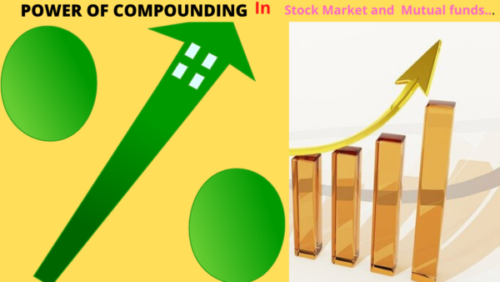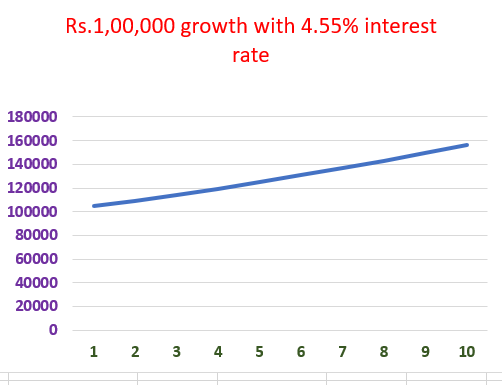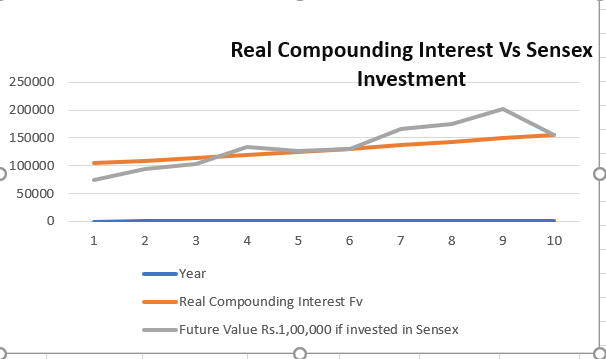# Power of Compounding – Why it is not there in (Mutual Funds), Stock market?The power of compounding exists in Mutual funds and in Stock Market is most people believe.

But actually, this compounding interest benefit is not there in mutual funds.

In addition, Many investors believing this wrong assumption and investing blindly in mutual funds, stock market.

In this article, I will showcase why there is no Power of Compound interest in the stock market and equity mutual funds.

What is  Compounding Interest.?…

Compounding interest means that you are getting interest on interest.

Let’s say you have invested in Bank Fd Rs.1,00,000, the bank promised you to pay 6% compound interest every year for a 10 years period.

If you see in the above image, the interest rate for every year is constant, and it is 4.55% per year.

In addition, You can see that the investment of Rs.1,00,000 lakh got an interest amount Rs.4550 and the Year-end value is Rs.1,04,550 ( Rs.1,00,000 + Rs.4,550).

But in the second year, You will get 4.55% interest on  Rs.1,0,4,550 not on Rs.1,00,000.

So, the interest payment in the second year is Rs.4,757, and 2nd year-end value is Rs.1,09,307 ( Rs.1,04,550 + Rs.4,757).

It is very clear that in the above image that your interest calculated every year on the previous year’s year-end value. In

As a result of this, you are earning interest on the previous year’s interest payment also.

In addition, you can find that the interest rate of 4.55% is constant all these 10 years in the above image.

You have invested Rs.1,00,000 @ 4,5%, and got Rs.1,04,550 after 1 year.

To compute we have to assume in the second year that you invested Rs.1,04,550 ( not Rs.1,00,000), and the interest payment should be calculated on Rs.1,04,550.You can see in the above that the investment has grown in straight line as the interest rate is constant.

If Compounding interest is not there and if there is only interest is there, then the values will be like shown in the below image.

You can clearly see in the above image that the interest amount is not added in the 2nd year and every year interest payment is constant ( Rs.4,550).

As a result of this total future value after 10 years in the above image is Rs.1,45,500.

While at the same in other images ( compound interest) the future value after 10 years is Rs.1,56,037.

So, it is clear that we will get more profit if there is compounding interest ( unlike in simple interest).

In addition, we understood that in the power of compounding the interest rate will be constant every year.

### Do the mutual funds and stock market have the power of Compounding Benefit?…

Now, Let’s take the last 10 years’ historical data of the Sensex and analyze it to know power of compounding benefit in the stock market.

You can see in the above image that the Sensex return is not constant every year.

In addition, some years the Sensex has given negative returns also.

We learned in earlier compounding interest example of bank fd that the return should be constant, and it should not be negative or low.

But in the above image, not even one parameter is matching.

In addition, the year 2020 is not yet over while writing this article. But I took the recent Sensex closing price for the purpose of calculating return.

Now let’s calculate the total future value of Rs.1,00,000 invented in Sensex with the above return on investment for 10 years.

If you see in the above the future value of 1 lakh if invested in Sensex for the last 10 years from 2011 to 2020 is Rs.1,56,028.

### What is the CAGR ( Compounding Interest ) in the above image?…

Actually, there is no compounding interest benefit in mutual funds, stock market.

But to understand the average profit % that we got from Sensex investment, we can calculate the CAGR of an investment.

CAGR = (Future Value/(Present Value)^(1/N)-1

If we calculate the CAGR of the Rs.1,00,000 investment using the above formulae, it is 4.55%.

Present Value = Rs.1,00,000

Future Value = Rs.1,56,028.

N                  = 10 years.

### But it is not a real compounding interest.

The real compounding interest will be constant every year and the future value should grow in a straight line if plot a line.

In addition, we should not get a loss in any year in a real Compounding Benefit scenario.

If we just use the CAGR formulae without using common sense, it will misguide us.In this article, we took interest rate in real compounding interest example as 4.55%.

If we calculate the CAGR ( not real CAGR) using formulae in Sensex investment for the last 10 years also we got the same return ( 4.55%) like in real compounding interest example.

But if you see in the above line chart, the real compounding line has grown smoothly, while at the same time the Sensex investment line has not grown in a straight line.

In addition, the 1 lakh investment gone into negative for 1st year and it is below the yellow line.

So, it is very clear that not only there is no power of compounding interest benefit in the stock market or mutual funds investment but also you may get a loss sometimes.

#### Conclusion About Power of Compounding in the Stock market and mutual funds…

In this article, we understood that there is no real power of compounding benefit in the stock market and mutual funds.

I have explained with different examples and shown the fact.

So, do not invest based on the belief that mutual funds and the stock market have compounding interest benefit.

In addition, You have to invest in the equity markets understanding the risks involved in it.

Otherwise, You may end up losing your invested capital also when you need the money.

Share With Friends and Family